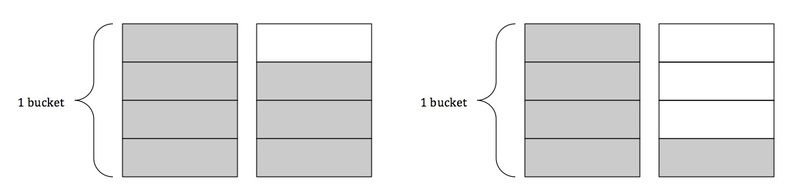# Plastic Building Blocks

Alignments to Content Standards: 4.NF.B.3.c

Dennis and Cody are building a castle out of plastic building blocks. They will need $2\frac12$ buckets of blocks for the castle they have in mind. Dennis used to have two full buckets of blocks but lost some and now has $1\frac34$ buckets. Cody used to have two full buckets of blocks too, but now has $1\frac14$ buckets. If Dennis and Cody combine their buckets of blocks, will they have enough to build their castle?

## IM Commentary

The purpose of this task is to have students add mixed numbers with like denominators. This task illustrates the different kinds of solution approaches students might take to such a task. Two general approaches should be anticipated: one where students calculate exactly how many buckets of blocks the boys have to determine an answer, and one where students compare the given numbers to benchmark numbers.

If students choose to approach the problem by calculating exactly how many buckets of blocks the boys have, at least two methods should be anticipated here as well. While some students will add the whole numbers together, then the fractions together, and finally combine the two, other students may choose to find equivalent fractions for the mixed numbers and then add the fractions. If students choose to approach the problem by comparing the given numbers to benchmarks, they are illustrating a strong number sense, although they may have some difficulty showing all of their work. Ideally in a classroom situation, some students will approach it via calculation and others will approach it via comparison, which could generate a very good classroom discussion about fraction number sense.

## Solutions

Solution: Drawing a picture

The picture on the left represents Dennis' buckets of building blocks, and the picture on the right represents Cody's buckets of building blocks.Combining the boys' buckets, that is pouring Cody's bucket that is not full into Dennis' bucket that is not full (or vice versa), we see thatthe boys have 3 full buckets (and one empty bucket).

Solution: Using properties of operations

We can approach the addition of mixed numbers in a couple of ways.

Since $1\frac34$=$1$+$\frac34$ and $1\frac14$=$1$+$\frac14$, $$1\frac34+1\frac14=1+\frac34+1+\frac14$$

By the commutative property of addition we have that

$$1+\frac34+1+\frac14=1+1+\frac34+\frac14$$

Now we can simply add the whole numbers together and add the fractions together.

Adding the whole numbers we have

$$1+1=2$$

$$\frac34+\frac14=\frac44=1$$

Together we have

$$2+1=3$$

Combined, the boys have 3 buckets of plastic building blocks.

Solution: Converting mixed numbers to equivalent fractions

We can record this symbolically as follows. Since:

\begin{align} 1\frac34 &= 1 + \frac34\\ &=\frac44 + \frac34\\ &=\frac74 \end{align}
and
\begin{align} 1\frac14 &= 1 + \frac14\\ &=\frac44 + \frac14\\ &=\frac54 \end{align}
We have that:
\begin{align} 1\frac34+1\frac14 &= \frac74+\frac54\\ &=\frac{12}{4}\\ &=3 \end{align}

Just as we saw in the picture, the boys have 3 buckets of plastic building blocks all together.

Solution: Estimating

To determine whether or not the boys have enough plastic building blocks to build their mansion we can compare the number of buckets they have to the minimum number of buckets they need. We don’t need to calculate the exact number of buckets they have but only whether they have more or less than $2\frac12$ buckets.

Dennis has $1\frac34$ buckets of plastic building blocks, and Cody has $1\frac12$ buckets of plastic building blocks. If we only count their full buckets, Dennis has $1$ bucket and Cody has $1$ bucket. Together, the boys have $2$ full buckets. They only need another $\frac12$ of a bucket to build their mansion. In addition to his $1$ full bucket, Dennis also has $\frac34$ of a bucket, which is more than $\frac12$ because $$\frac34\gt\frac24=\frac12$$

Dennis and Cody have $2$ full buckets of blocks and more than $\frac12$ of an additional bucket of blocks, meaning that they have more than $2\frac12$ buckets of blocks. The answer is yes; the boys will have enough plastic building blocks to build the mansion they have in mind.